What is the electron dot diagram for an oxygen atom?Coco

Concept:

The distribution of electrons in different orbitals is called electronic configuration. The electrons filled in the outermost shell are called valence electrons.

Electron dot diagram or Lewis dot diagram is a representation of an atom in which the valence electrons are shown as dot around the letter symbol of the element.

Solution:

Step 1: The letter symbol for oxygen is ‘O’.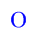Step 2: The electronic configuration of oxygen is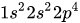. The electrons present in the highest principal energy level are valence electrons. The number of valence electrons for oxygen atom is 6.

Step 3: Distribute the valence electrons around the oxygen atom.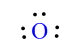Similar problems:

Question:

What is the electron dot diagram for carbon atom?

Solution:

Step 1: The letter symbol for carbon is ‘C’.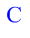Step 2: The electronic configuration of carbon is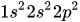. The electrons present in the highest principal energy level are valence electrons. The number of valence electrons for carbon atom is 4.

Step 3: Distribute the valence electrons around a carbon atom.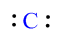Question:

What is the electron dot diagram for nitrogen atom?

Solution:

Step 1: The letter symbol for carbon is ‘N’.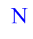Step 2: The electronic configuration of nitrogen is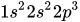. The electrons present in the highest principal energy level are valence electrons. The number of valence electrons for nitrogen atom is 5.

Step 3: Distribute the valence electrons around the nitrogen atom.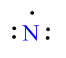Need homework help now?

tutors online

Related Questions
2. (11 marks) a) At 400 °C, ammonium iodide decomposes in the following endothermic reaction: NH41(s) + NH3(g) + HI(g) Kp = 0.215 at 400 °C. Write the equilibrium expression for this reaction. 15.0 g of NH4I was placed in an empty 3.0 L flask and heated to 400 °C. What is the partial pressure of NH3 in the flask once the reaction has reached equilibrium? How would the following changes to the equilibrium mixture affect the number of moles of NH3 in the flask and the value of K, once equilibrium is re-established? (Use increase, decrease or stay the same.) Change Effect on moles of NH3 Ke value Increase temperature Increase volume of flask Remove half of HI gas
Notes
Me

OR

Don't have an account?

Join OneClass

Access over 10 million pages of study
documents for 1.3 million courses.

Join to view

OR

By registering, I agree to the Terms and Privacy Policies
Just a few more details

So we can recommend you notes for your school.